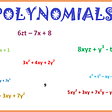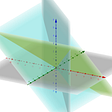# A look at the Collatz conjecture

The Collatz conjecture is like a lot of other conjectures in mathematics, in that it is very easy to explain but seems difficult and perhaps impossible to prove or disprove.

Pick an integer. If it’s even, halve it. For example, pick 26. Half of that is 13. If the number is odd, triple it and add 1. So thrice 13 is 39 and adding 1 gives us 40. Since 40 is even, we halve it, getting 20, which is also even, so we halve again, and again, getting 10 and 5.

And thrice 5 plus 1 is 16, after which several consecutive halving steps gets us down to 1, and from there to the infinite cycle 4, 2, 1.

The Collatz conjecture states that if you pick a positive integer, enough iterations of the Collatz function will always lead to 1.

What makes it so difficult to prove is that in almost every case it’s hard to know how many iterations of the Collatz function are necessary without actually going through the iterations.

If you pick a power of 2, then obviously it’s going to reach 1 after as many halving steps as the exponent indicates, e.g., 2¹⁰ will reach 1 in ten steps.

Given that iterating the Collatz function from 2¹⁰ = 1024 reaches 1 in ten steps, can you guess how many steps it takes starting from 1023 or 1025? Without doing the calculations, I can only tell you that it takes more than ten steps, but I can only guess how many more.

Better to have my computer do the calculations. I wrote a Scala program for this purpose, you can use Python or whatever you like. I’m not going to go into the computer programming side of it for this article.

So with the program loaded in, my computer tells me it takes sixty-two iterations of the Collatz function to go from 1023 to 1, and just thirty-six iterations to go from 1025 to 1. Contrast that to the smaller number 864, which takes more than a hundred iterations to reach 1.

If the Collatz conjecture is true, every positive integer can be placed on a tree with 1 at the root, though some small numbers might be at a very great distance from 1, a distance greater than that of some much larger numbers. But if the conjecture is false, then there are positive integers that have no path connecting them to 1.

Note that the conjecture specifies positive numbers. It’s obviously false for negative numbers. For example, −1024 reaches −1, not 1, in ten halving steps. We could make an equivalent conjecture for negative integers, that they all eventually reach −1.

But that conjecture is quickly proven false. Trying −7, we get: −7, −20, −10, −5, −14, −7, −20, −10, −5, −14, −7, −20, −10, −5, −14, etc. Plenty other negative numbers, like −28, −56, −112, etc., eventually lead into that cycle.

So if the Collatz conjecture is false, it’s almost certainly because there are some positive values of n that lead to a cycle of positive numbers like the cycle of negative numbers we saw with −7.

The Collatz conjecture has been checked well beyond 2⁶⁴, which is a number of significance to any computer programmer hoping to check numbers that haven’t been checked before.

In mathematics, no abundance of examples is sufficient to prove a conjecture, but a single counterexample is enough to disprove a conjecture. If the conjecture has been verified to hold up to 2⁷² + 1, it could very well be the case that 2⁷² + 3 is a counterexample.

Obviously we can’t examine every positive integer. But mathematicians have been able to prove lots of things about infinitely many cases without ever examining infinitely many cases.

For example, suppose that you just noticed that 2² − 1 = 3 is prime, and you wonder if there are other primes of the form n² − 1, where n is an integer. There are not. This is a well-known fact, and if you don’t know the proof, you can easily obtain it yourself.

You can’t look at every possible value of n, but if you look at a few small values of n you might notice what you need for a proof. Notice that 15 = 3 × 5, 35 = 5 × 7, 63 = 7 × 9.

This suggests that n² − 1 = (n − 1)(n + 1). Maybe you remember FOIL (first, outer, inner, last) from high school algebra. Applying FOIL, we see that (n − 1)(n + 1) = n² + nn − 1. Obviously −n cancels out +n. So we’re left with n² − 1. From there only a little work is needed to arrive at a mathematically rigorous proof.

If the proof of the Collatz conjecture was that simple, it would have been found by now. Still, it sure is interesting to examine this conjecture.

--

--

--

## More from Alonso Del Arte

is a composer and photographer from Detroit, Michigan. He has been working on a Java program to display certain mathematical diagrams.

Love podcasts or audiobooks? Learn on the go with our new app.

## Let’s break the encryption## The Journey of Mathematical Ideas## Math for artists: challenge 2 — aspect ratio tool and House of Wisdom## Gamma transforms: How to hedge squeeth using Uni V3## Getting Down with Fermat## Experimental Math — Computing Units of Modular Rings## Alonso Del Arte

is a composer and photographer from Detroit, Michigan. He has been working on a Java program to display certain mathematical diagrams.

## Polynomials Class 10th## Linear Algebra II## Curve Sketching## My crown jewels in Mathematics…